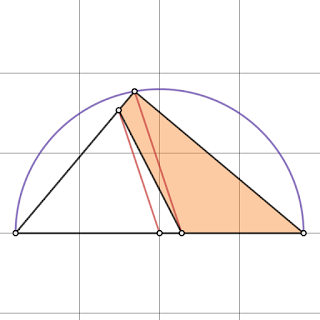## onsdag den 24. april 2019

### Top 10.23

herfraOrange Fraction = ?BD / AB = ? ( Pool Table )

If Blue Area = Orange Area, then Coloured Fraction = ?
Blue Area = ?
Blue Area = ?
If Angle SAT = 30, then r = ?
Blue Area = ?
Orange Fraction = ?
Area Orange minus Area Blue = 0
White Area = ?

## søndag den 21. april 2019

### Top 10.22

Area Yellow = ?
What is X = ?
Green Area = ?
Area BGEH = ?
Tangent AP = ?
Does it ever sink into a Hole = ?
Orange Area = ?
What is the Radius = ?

## torsdag den 18. april 2019

### Top 10.21

Ln x ( Differentialregning )
Area of Red Triangle = ?
Area of Triangle ABC divided by Area of Arc ABC = ?
Triangle Area = ?
Area Green = ?
X = DH = ?
Angle OQP = ?
Blue Part of Total Area = ?
Area of Square ABCD = ? ( Try making R a Slider Value between 5 and 8 )

## tirsdag den 16. april 2019

### Top 10.20

BM = ? ( Similar Triangles )
EG = ?
Orange Part of Total Area = ?
Circle in an Ellipse with Tangent
Area of Square = ?
FB / FA = ?
X = CK = ?
Angle CBD = ?
Angle CBE = ?
Area of Larger Square = ?

## mandag den 15. april 2019

### Top 10.19

Area of Hexagon = ?
Fraction Blue = ?
Purple Fraction = ? ( Similar Triangles )
Tan RAT = ?
Area ABCD = ?
Area of Semicircle = ?
If S = 1 then Shaded Area = ?
Geneva Drive Step 6
Orange Fraction = ?
Circles and 3-4-5 Triangle

## torsdag den 11. april 2019

### Top 10.18

Blue / Orange = ?
Area Red - Area White = ?
In a 3-4-5 Trangle R of a Semicircle = ?
Radius of Orange Circle = ?
Area of Orange Square = ?
Area ABCD = ?
Area of Orange Square = ?
Area Orange = ?

## tirsdag den 9. april 2019

### Top 10.17

Fraction Red = ?
Area Green / Area Blue = ?
BC = AB = AC = ? ( Similar Triangles )
X = BD = ?
Area Blue = ?
Angle APD = ?
Angle BAE = ?
Minimum of AB = ?
Angle BAE = ?
X / Y = ?

## mandag den 8. april 2019

### Top 10.16

Area Black = ?
Side s = 2 ⇒ Area orange = ?
Angle ABD = ?
DP = 15 ⇒ Diameter d = ?
BP / PT = ½ ⇒ tan BCA = ?
Orange Fraction = ?
Angle BED = ?
R / r = O₂ / o₂ = ?
Scale Factor SF = ?

## torsdag den 4. april 2019

### Top 10.15

Area Blue / Area Orange = ?
Area Orange = ?
Area Orange = ?
X / R = ? ( Equal Perimeters )
Area Orange / Area Purple = ?
Angle BPC = ? ( Similar Triangles )
Angle ACF = ?
Angle CBD = ?
Angle ABC = ?
Perimeter of APT = ? ( Similar Triangles )